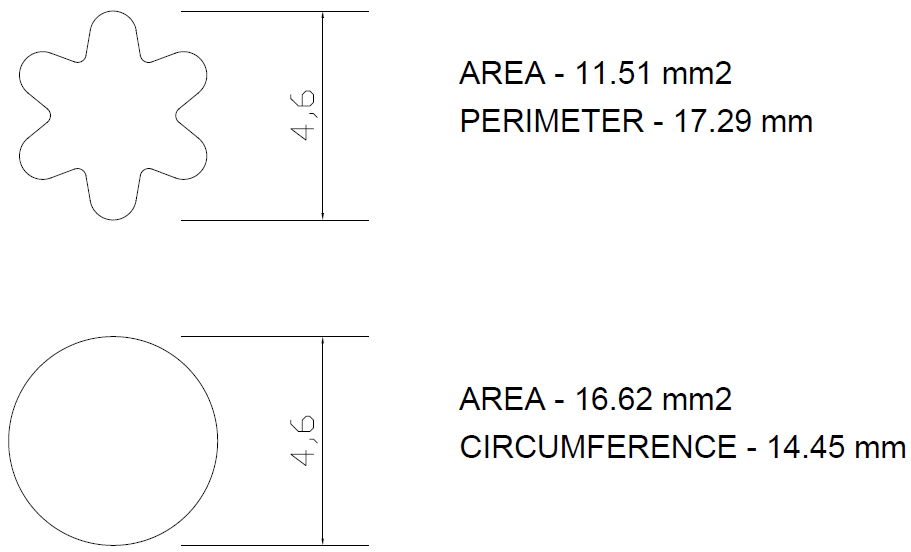# Ceramic Membranes – Star v Circular Channel

Mantec’s Star-Sep™ Membrane filter has been specifically developed for efficient crossflow microfiltration. The filter channel’s unique ‘star’ form increases the filtration area and induces turbulence at lower crossflow velocities. Not only does this lower the volume compared with a circular channel of the same diameter, but also results in a reduction of the pumping energy requirement. The cost effectiveness of the process is therefore substantially improved.

A crossflow velocity in the region of 2 to 6 m/s is generally considered necessary to provide the surface shear on the membrane to break up the fouling layer. The optimum velocity depends on the product being processed.

#### Diameter/Circumference Relationship – Tubular Membranes

With a constant crossflow velocity, it is beneficial to design a system in which the channels have the following characteristics:

• maximum circumference / perimeter (for greater filtration area), and
• minimum cross-sectional area (for lower retentate flow and hence lower energy)

Consider a circular channel having a diameter of 4.6mm and a star-shaped channel having an equivalent outer tip diameter (Fig. 1).Fig. 1 – circular and star-shaped channels of equivalent outer diameter

#### Filtration Area

Considering a 19 channel x 1.2m long membrane, the filtration area is as follows:

Filtration Area = Perimeter of Channel x Length x No. of Channels.

• Filtration Area (Circular Channel) m2 = 0.01445 x 1.2 x 19 = 0.329 m2
• Filtration Area (Star Channel) m2 = 0.01729 x 1.2 x 19 = 0.394 m2

The perimeter of the star channel (and hence filtration area) is 20% greater than that of the equivalent circular channel.

#### Cross-Sectional Area / Crossflow Volumetric Flow

The crossflow volumetric flow is proportional to the cross-sectional area of the flowpath. Therefore, to reduce the crossflow volume (and hence the pumping energy), whilst maintaining the velocity, the appropriate variable to decrease is the cross-sectional area of the flowpath.

Crossflow Volumetric Flow (m3/hr) =
cross sectional area (m2) x velocity (m/s) x 3600 x number of channels

Circular Channel
Vol. Flow (m3/hr) = 0.00001662 (m2) x 4 (m/s) x 3600 x 19 = 4.55 (m3/hr)

Star Channel
Vol. Flow (m3/hr) = 0.00001151 (m2) x 4 (m/s) x 3600 x 19 = 3.15 (m3/hr)

The cross sectional area of star is 30% less than that of the equivalent circular channel. This results in a corresponding 30% less volumetric flow required to achieve the same velocity.